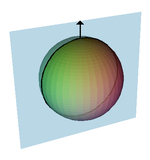# Henagon

﻿
Henagon
HenagonOn a circle, a henagon is a tessellation with a single vertex, and one 360 degree arc.
Edges and vertices 1
Schläfli symbol {1}
Coxeter–Dynkin diagramsInternal angle (degrees) 360°

In geometry a henagon (or monogon) is a polygon with one edge and one vertex. It has Schläfli symbol {1}. Since a henagon has only one side and only one interior angle, every henagon is regular by definition.

## In Euclidean geometry

In Euclidean geometry a henagon is usually considered to be an impossible object, because its endpoints must coincide, unlike any Euclidean line segment. For this reason, most authorities do not consider the henagon as a proper polygon in Euclidean geometry.

## In spherical geometry

In spherical geometry, on the other hand, a finite henagon can be drawn by placing a single vertex anywhere on a great circle. Two henagons can be used to construct a dihedron on a sphere, with Schläfli symbol, {1,2}.Henagonal dihedron (up-arrow represents vertex).

The henagon can be used in spherical polyhedra, for example the henagonal dihedron {1, 2}, the digonal hosohedron {2, 1} and the henagonal henahedron {1, 1}. The henagonal henahedron consists of a single vertex, no edges and a single face (the whole sphere minus the vertex.)